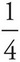# AP Physics 1 Question 203: Answer and Explanation

### Test Information

Question: 203

3. A frictionless inclined plane of length 20 m has a maximum vertical height of 5 m. If an object of mass 2 kg is placed on the plane, which of the following best approximates the net force it feels?

• A. 5 N
• B. 10 N
• C. 15 N
• D. 20 N

The net force that the object feels on the inclined plane is mg sin θ, the component of the gravitational force that is parallel to the ramp. Since sin θ = (5 m)/(20 m) =, you get Fnet = (2 kg)(10 N/kg)() = 5 N.